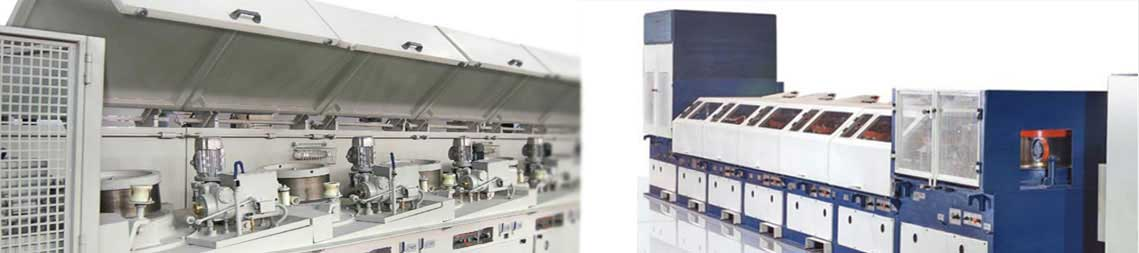# Formulas

Our designing team uses various wire drawing formulas for designing a variety of wires with our wire drawing machines such as weight / length relationship formula, surface area formula, zinc coating formula and formula for reduction in area

 FORMULA ONE FORMULA TWO Weight / Length Relationship: The weight per foot of wire is : (2)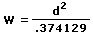Example : The Weight per foot os .090" wire is :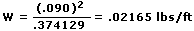The length per lb of wire is: (3)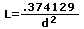Where: w = weight per foot (lbs/ft) d = wire diameter(inch) L = length per lb (ft/lb) Example : The length per lb of .090" wire is: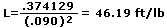* Surface Area: The surface area of one foot of wire is: (4) a = X 0.090 / 12 = 0.0236 ft2/ft The surface area of one lb of wire is : (5) a/w = 1 / (36 X d X y) Where: a = surface area of one foot of wire (ft2/ft) a/w = surface area of one lb of wire (ft2/lb) d = wire diameter (inch) w = weight of one foot of wire (lb/ft) y = specific weight (for steel, 0.283 lbs/in3) Example : The surface are of one lb of 0.090" diameter wire is : a/w = 1 / (36 X 0.090 X 0.2836) = 1.088 ft2lb * Refer to Page 369, Volume 2, Steel Wire Handbook

 FORMULA THREE FORMULA FOUR ZINC COATING The Percent zinc coating on d" diameter wire coated with coz/ft2zinc is: (6)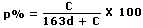Example : for 0.090" diameter wire coated 0.3 oz/ft2 : (6)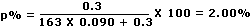The weight of zinc on d" diameter wire coated with p% zinc is : (7)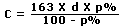Where : p% = weight percent of zinc(%) c = zinc coating weight (oz/ft2) d = wire diameter(inch) Example: for 0.090" diameter wire, 2.00% zinc coating weights :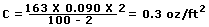* Refer to Page 301, Volume 1, Steel Wire Handbook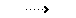Graph for Formula Four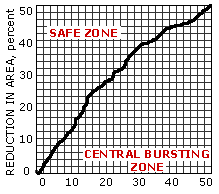INCLUDING DIE ANGLE, degrees Work Sheet for designing tooling geometry to prevent central bursting. Reduction in Area: Step reduction, drawing wire from di-1to di (8)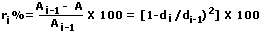Examples :drawing from step 3 to step 4: r4[1-(0.090/0.102)2] X 100 drawing 0.102" wire to 090" r = [1-(0.090/0.102)2 X 100 = 22.15% Total Reduction, drawing from d0 to di (9)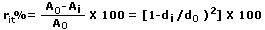Examples :at step 4: r = [1-(d4/d0)2] X 100 drawing 7/32" rod to 0.102": r = [1 - (0.102/0.219)2] X 100 = 78.31% Overall reduction, drawing from d0 to df (10)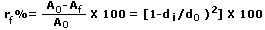Examples :drawing 7/32" to 0.090" : rf= [1-(0.090/0.219)2] X 100 = 83.11% NOTES : 1. Reduction in area is not additive, rf is not f1 + r2 + ... + rn 2. Reduction is always positive. 3. Reduction is never larger than 100%. 4. For ferrous wire, step reduction is usually between 7% and 38% (limits depend on the product). 5. For overall reduction greater than 85%, the process has to be carefully designed. 6. Clue : for final diameter of one half the original, reduction is 75%. To Compute the diameter from reduction : (11)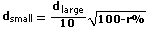Example:Drawing 7/32" rod 25% reduction: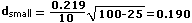Given the small diameter, the large diameter is: (12)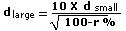Where: r = drawing reduction in area(%) d = wire diameter(inch) Example: For an overall reduction of 83.1%, an 0.090" wire will require a starting diameter of: do = The Required Percent Reduction Between the Bottom and Top Block of a Double-Draw Single-Block Frame: Total required reduction at the second hole (13) = 100% -